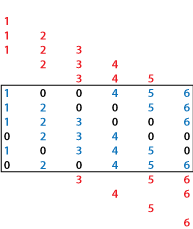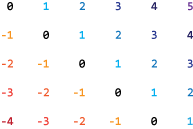# spdiags

Extract nonzero diagonals and create sparse band and diagonal matrices

## Syntax

``Bout = spdiags(A)``
``[Bout,id] = spdiags(A)``
``Bout = spdiags(A,d)``
``S = spdiags(Bin,d,m,n)``
``S = spdiags(Bin,d,A)``

## Description

example

````Bout = spdiags(A)` extracts the nonzero diagonals from `m`-by-`n` matrix `A` and returns them as the columns in `min(m,n)`-by-`p` matrix `Bout`, where `p` is the number of nonzero diagonals.```

example

````[Bout,id] = spdiags(A)` also returns the diagonal numbers `id` for the nonzero diagonals in `A`. The size of `Bout` is `min(m,n)`-by-`length(id)`.```

example

````Bout = spdiags(A,d)` extracts the diagonals in `A` specified by `d` and returns them as the columns of `min(m,n)`-by-`length(d)` matrix `Bout`.```

example

````S = spdiags(Bin,d,m,n)` creates an `m`-by-`n` sparse matrix `S` by taking the columns of `Bin` and placing them along the diagonals specified by `d`.```

example

````S = spdiags(Bin,d,A)` replaces the diagonals in `A` specified by `d` with the columns of `Bin`.```

## Examples

collapse all

Create a tridiagonal matrix using three vectors, change some of the matrix diagonals, and then extract the diagonals.

Create a 9-by-1 vector of ones, and then create a tridiagonal matrix using the vector. View the matrix elements.

```n = 9; e = ones(n,1); A = spdiags([e -2*e e],-1:1,n,n); full(A)```
```ans = 9×9 -2 1 0 0 0 0 0 0 0 1 -2 1 0 0 0 0 0 0 0 1 -2 1 0 0 0 0 0 0 0 1 -2 1 0 0 0 0 0 0 0 1 -2 1 0 0 0 0 0 0 0 1 -2 1 0 0 0 0 0 0 0 1 -2 1 0 0 0 0 0 0 0 1 -2 1 0 0 0 0 0 0 0 1 -2 ```

Change the values on the main (`d = 0`) diagonal of `A`.

```Bin = abs(-(n-1)/2:(n-1)/2)'; d = 0; A = spdiags(Bin,d,A); full(A)```
```ans = 9×9 4 1 0 0 0 0 0 0 0 1 3 1 0 0 0 0 0 0 0 1 2 1 0 0 0 0 0 0 0 1 1 1 0 0 0 0 0 0 0 1 0 1 0 0 0 0 0 0 0 1 1 1 0 0 0 0 0 0 0 1 2 1 0 0 0 0 0 0 0 1 3 1 0 0 0 0 0 0 0 1 4 ```

Finally, recover the diagonals of `A` as the columns in a matrix.

```Bout = spdiags(A); full(Bout)```
```ans = 9×3 1 4 0 1 3 1 1 2 1 1 1 1 1 0 1 1 1 1 1 2 1 1 3 1 0 4 1 ```

Extract the nonzero diagonals of a matrix and examine the output format of `spdiags`.

Create a matrix containing a mix of nonzero and zero diagonals.

```A = [0 5 0 10 0 0 0 0 6 0 11 0 3 0 0 7 0 12 1 4 0 0 8 0 0 2 5 0 0 9];```

Extract the nonzero diagonals from the matrix. Specify two outputs to return the diagonal numbers.

`[Bout,d] = spdiags(A)`
```Bout = 5×4 0 0 5 10 0 0 6 11 0 3 7 12 1 4 8 0 2 5 9 0 ```
```d = 4×1 -3 -2 1 3 ```

The columns of the first output `Bout` contain the nonzero diagonals of `A`. The second output `d` lists the indices of the nonzero diagonals of `A`. The longest nonzero diagonal in `A` is in column 3 of `Bout`. To give all columns of `Bout` the same length, the other nonzero diagonals of `A` have extra zeros added to their corresponding columns in `Bout`. For `m`-by-`n` matrices with `m < n`, the rules are:

• For nonzero diagonals below the main diagonal of `A`, extra zeros are added at the tops of columns (as in the first two columns of `Bout`).

• For nonzero diagonals above the main diagonal of `A`, extra zeros are added at the bottoms of columns (as in the last column of `Bout`).

`spdiags` pads `Bout` with zeros in this manner even if the longest diagonal is not returned in `Bout`.

Create a 5-by-5 random matrix.

`A = randi(10,5,5)`
```A = 5×5 9 1 2 2 7 10 3 10 5 1 2 6 10 10 9 10 10 5 8 10 7 10 9 10 7 ```

Extract the main diagonal, and the first diagonals above and below it.

```d = [-1 0 1]; Bout = spdiags(A,d)```
```Bout = 5×3 10 9 0 6 3 1 5 10 10 10 8 10 0 7 10 ```

Try to extract the fifth super-diagonal (`d = 5`). Because `A` has only four super-diagonals, `spdiags` returns the diagonal as all zeros of the same length as the main (`d = 0`) diagonal.

`B5 = spdiags(A,5)`
```B5 = 5×1 0 0 0 0 0 ```

Examine how `spdiags` creates diagonals when the columns of the input matrix are longer than the diagonals they are replacing.

Create a 6-by-7 matrix of the numbers 1 through 6.

`Bin = repmat((1:6)',[1 7])`
```Bin = 6×7 1 1 1 1 1 1 1 2 2 2 2 2 2 2 3 3 3 3 3 3 3 4 4 4 4 4 4 4 5 5 5 5 5 5 5 6 6 6 6 6 6 6 ```

Use `spdiags` to create a square 6-by-6 matrix with several of the columns of `Bin` as diagonals. Because some of the diagonals only have one or two elements, there is a mismatch in sizes between the columns in `Bin` and diagonals in `A`.

```d = [-4 -2 -1 0 3 4 5]; A = spdiags(Bin,d,6,6); full(A)```
```ans = 6×6 1 0 0 4 5 6 1 2 0 0 5 6 1 2 3 0 0 6 0 2 3 4 0 0 1 0 3 4 5 0 0 2 0 4 5 6 ```

Each of the columns in `Bin` has six elements, but only the main diagonal in `A` has six elements. Therefore, all the other diagonals in `A` truncate the elements in the columns of `Bin` so that they fit on the selected diagonals:The way `spdiags` truncates the diagonals depends on the size of `m`-by-`n` matrix `A`. When $\mathit{m}\ge \mathit{n}$, the behavior is as pictured above:

• Diagonals below the main diagonal take elements from the tops of the columns first.

• Diagonals above the main diagonal take elements from the bottoms of columns first.

This behavior reverses when $\mathit{m}<\mathit{n}$:

```A = spdiags(Bin,d,5,6); full(A)```
```ans = 5×6 1 0 0 1 1 1 2 2 0 0 2 2 3 3 3 0 0 3 0 4 4 4 0 0 5 0 5 5 5 0 ```• Diagonals above the main diagonal take elements from the tops of the columns first.

• Diagonals below the main diagonal take elements from the bottoms of columns first.

## Input Arguments

collapse all

Input matrix. This matrix is typically (but not necessarily) sparse.

Data Types: `single` | `double` | `int8` | `int16` | `int32` | `int64` | `uint8` | `uint16` | `uint32` | `uint64` | `logical`
Complex Number Support: Yes

Diagonal numbers, specified as a scalar or vector of positive integers. The diagonal numbers follow the same conventions as `diag`:

• `d < 0` is below the main diagonal, and satisfies ```d >= -(m-1)```.

• `d = 0` is the main diagonal.

• `d > 0` is above the main diagonal, and satisfies ```d <= (n-1)```.

An `m`-by-`n` matrix `A` has `(m + n - 1)` diagonals. These diagonals are specified in the vector `d` using indices from `-(m-1)` to `(n-1)`. For example, if `A` is 5-by-6, it has 10 diagonals, which are specified in the vector `d` using the indices -4, -3 , ... 4, 5. The following diagram illustrates this diagonal numbering.If you specify a diagonal that lies outside of `A` (such as `d = 7` in the example above), then `spdiags` returns that diagonal as all zeros.

Example: `spdiags(A,[3 5])` extracts the third and fifth diagonals from `A`.

Diagonal elements, specified as a matrix. This matrix is typically (but not necessarily) full. `spdiags` uses the columns of `Bin` to replace specified diagonals in `A`. If the requested size of the output is `m`-by-`n`, then `Bin` must have `min(m,n)` columns.

With the syntax `S = spdiags(Bin,d,m,n)`, if a column of `Bin` has more elements than the diagonal it is replacing, and `m >= n`, then `spdiags` takes elements of super-diagonals from the lower part of the column of `Bin`, and elements of sub-diagonals from the upper part of the column of `Bin`. However, if `m < n` , then super-diagonals are from the upper part of the column of `Bin`, and sub-diagonals from the lower part. For an example of this behavior, see Columns and Diagonals of Different Sizes.

Data Types: `single` | `double` | `int8` | `int16` | `int32` | `int64` | `uint8` | `uint16` | `uint32` | `uint64` | `logical`
Complex Number Support: Yes

Dimension sizes, specified as nonnegative scalar integers. `spdiags` uses these inputs to determine how large a matrix to create.

Example: `spdiags(Bin,d,300,400)` creates a 300-by-400 matrix with the columns of `B` placed along the specified diagonals `d`.

## Output Arguments

collapse all

Diagonal elements, returned as a full matrix. The columns of `Bout` contain diagonals extracted from `A`. Any elements of `Bout` corresponding to positions outside of `A` are set to zero.

Diagonal numbers, returned as a column vector. See `d` for a description of the diagonal numbering.

Output matrix. `S` takes one of two forms:

• With `S = spdiags(Bin,d,A)`, the specified diagonals in `A` are replaced with the columns in `Bin` to create `S`.

• With `S = spdiags(Bin,d,m,n)`, the `m`-by-`n` sparse matrix `S` is formed by taking the columns of `Bin` and placing them along the diagonals specified by `d`.

## Version History

Introduced before R2006a